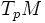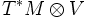# Vector space-valued differential 1-form

## Definition

### Symbol-free definition

A vector space-valued differential 1-form on a differential manifold, is defined in the following ways:

• It is a smooth map from the tangent bundle of the manifold, to a given vector space, such that the restriction to the tangent space at any point, is a linear map
• It is a smoothly varying collection of linear maps from the tangent spaces at different points on the manifold, to a fixed vector space
• It is a section of the tensor product of the cotangent bundle of the manifold, with a vector space

### Definition with symbols

Let$M$ be a differential manifold and$V$ a finite-dimensional real vector space. A$V$-valued differential 1-form on$M$ is defined as follows:

• A smooth map from$TM$ to$V$, whose restriction to each fiber$T_pM$, is a linear map from$T_pM$ to$V$
• A smoothly varying collection of linear maps from$T_pM$ to$V$ for every$p \in M$
• A section of the bundle$T^*M \otimes V$

A particular case of this is a Lie algebra-valued differential 1-form. Here, a Lie group$G$ is acting on the differential manifold$M$, and the vector space is the Lie algebra$\mathfrak{g}$ of$G$. Examples are the Maurer-Cartan form and connection form.